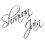# Is 2=1 Possible?

Once my teacher gave a proof of 2=1 which is as follow:

$${ 1 }^{ 2 }\quad =\quad 1\\ { 2 }^{ 2 }\quad =\quad 2\quad +\quad 2\\ { 3 }^{ 2 }\quad =\quad 3\quad +\quad 3\quad +\quad 3\\ .\\ .\\ .\\ .\\ .\\ { x }^{ 2 }\quad =\quad x\quad +\quad x\quad +\quad x\quad +\quad ........\quad upto\quad x\quad terms.\\ \\ Now\quad differentiating\quad both\quad sides\quad w.r.t\quad x,\\ 2x\quad =\quad 1\quad +\quad 1\quad +\quad 1\quad +\quad ........\quad upto\quad x\quad terms\\ ie,\quad 2x\quad =\quad x\\ \quad \quad \boxed { { 2\quad =\quad 1 } }$$

Now we were challenged to find the flaw in this proof ( Which we won )

Can you find the flaw in this proof ???Note by Shravan Jain
7 years, 5 months ago

This discussion board is a place to discuss our Daily Challenges and the math and science related to those challenges. Explanations are more than just a solution — they should explain the steps and thinking strategies that you used to obtain the solution. Comments should further the discussion of math and science.

When posting on Brilliant:

• Use the emojis to react to an explanation, whether you're congratulating a job well done , or just really confused .
• Ask specific questions about the challenge or the steps in somebody's explanation. Well-posed questions can add a lot to the discussion, but posting "I don't understand!" doesn't help anyone.
• Try to contribute something new to the discussion, whether it is an extension, generalization or other idea related to the challenge.

MarkdownAppears as
*italics* or _italics_ italics
**bold** or __bold__ bold
- bulleted- list
• bulleted
• list
1. numbered2. list
1. numbered
2. list
Note: you must add a full line of space before and after lists for them to show up correctly
paragraph 1paragraph 2

paragraph 1

paragraph 2

[example link](https://brilliant.org)example link
> This is a quote
This is a quote
    # I indented these lines
# 4 spaces, and now they show
# up as a code block.

print "hello world"
# I indented these lines
# 4 spaces, and now they show
# up as a code block.

print "hello world"
MathAppears as
Remember to wrap math in $$ ... $$ or $ ... $ to ensure proper formatting.
2 \times 3 $2 \times 3$
2^{34} $2^{34}$
a_{i-1} $a_{i-1}$
\frac{2}{3} $\frac{2}{3}$
\sqrt{2} $\sqrt{2}$
\sum_{i=1}^3 $\sum_{i=1}^3$
\sin \theta $\sin \theta$
\boxed{123} $\boxed{123}$

Sort by:

$Since\quad x\quad can\quad take\quad only\quad positive\quad integral\quad values\quad \\ (as\quad { -1 }^{ th }\quad term,\quad { 0 }^{ th }\quad term,\quad { 0.5 }^{ th }\quad term\quad does\quad not\quad exists),\quad \\ the\quad function\quad { x }^{ 2 }\quad is\quad discontinuous\quad \\ and\quad thus\quad is\quad non\quad differentiable.$

- 7 years, 5 months ago

Thank you for posting this question.

You're trying to use the equality:

${x}^{2} = x + x + ... + x$

You are trying to differentiate two functions. Let's see what we know about them.

Let $f(x)={x}^{2}$. The domain for this function is clearly $R$. And yeah when we differentiate we get $f'(x)=2x$.

Let $g(x)= x + x + ... + x$ (for the equality to be true x repeats in the sum exactly x times), then since a number can't repeat itself $\sqrt { 2 }$ times the domain of this function is $N$.

Now you differentiate. Tell me how you can calculate:

$g'\left( { x }_{ 0 } \right) =\lim _{ x\rightarrow { x }_{ 0 } }{ \frac { g\left( x \right) -g\left( { x }_{ 0 } \right) }{ x-{ x }_{ 0 } } }$

But you can't approach ${ x } _ { 0 }$ on $N$. You can only differentiate on intervals or $R$.

More than this the differentiation gives you the slope of the tangent in ${ x } _ { 0 }$, but you can't have a slope to a function that's made of dots.

Even if we took $f$ defined on $N$ we still wouldn't have been able to differentiate it either.

So no, 2 isn't 1.

- 7 years, 3 months ago

Well the you only differentiated the x's,the number of x's should also be differentiated as it is also a variable

- 7 years, 5 months ago

Since this function is non differentiable , there is no question of differentiating the no. of x's

- 7 years, 5 months ago

Well,I dint even consider that lol

- 7 years, 4 months ago